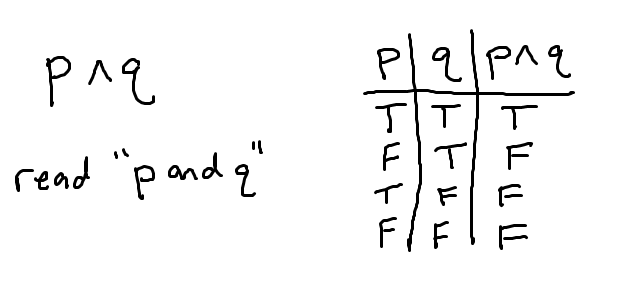# Truth tables – negation, conjunction, disjunction (“not”, “and”, “or”)

Truth tables are a way of analyzing how the validity of statements (called propositions) behave when you use a logical “or”, or a logical “and” to combine them. Propositions are either completely true or completely false, so any truth table will want to show both of these possibilities for all the statements made.

For all these examples, we will let p and q be propositions. They could be statements like “I am 25 years old” or “it is currently warmer than 70°”. Any statements that are either true or false.

## Negation – “not p”Negation is the statement “not p”, denoted $$\neg p$$, and so it would have the opposite truth value of p. If p is true, then $$\neg p$$ if false. If p is false, then $$\neg p$$ is true. Notice that the truth table shows all of these possibilities.

## Conjunction – “and”Consider the statement “p and q”, denoted $$p \wedge q$$. To analyze this, we first have to think of all the combinations of truth values for both statements and then decide how those combinations influence the “and” statement. In words:

• Row 1: the two statements could both be true.
In this case, it would make sense that “p and q” is also a true statement.

• Row 2: p could be false while q is true.
For “p and q” to be true, we would need BOTH statements to be true. Since one is false, “p and q” is false.

• Row 3: p could be true while q is false.
If this is the case, then by the same argument in row 2, “p and q” is false.

• Row 4: the two statements could both be false.
If both statements are false, then “p and q” is false.

The order of the rows doesn’t matter – as long as we are systematic in a way so that we do not miss any possible combinations of truth values for the two original statements p, q.

## Disjunction – “or”You may not realize it, but there are two types of “or”s. There is the inclusive or where we allow for the fact that both statements might be true, and there is the exclusive or, where we are strict that only one statement or the other is true. In math, the “or” that we work with is the inclusive or, denoted $$p \vee q$$. When we want to work with the exclusive or, we are specific and use different notation (you can read about this here: the exclusive or). This shows in the first row of the truth table, which we will now analyze:

• Row 1: the two statements could both be true.
Since we are working with the inclusive or, the statement “p or q” will be true in this case.

• Row 2: p could be false while q is true.
This is the essence of or. We are saying “one or both of the statements is true”. Therefore, “p or q” is true in this case.

• Row 3: p could be true while q is false.
Same idea as the second row.

• Row 4: the two statements could both be false.
Considering the meaning of or, if both statements are false, then it is not true that “p or q”, thus we list false for this statement.

## Summary

To keep track of how these ideas work, you can remember the following:

• “not p” always has the opposite truth value of p
• “p and q” is true only when both statements are true (false otherwise)
• “p or q” is false only when both statements are false (true otherwise)

Understanding these truth tables will allow us to later analyze complex compound compositions consisting of and, or, not, and perhaps even a conditional statement, so make sure you have these basics down!